# Dielectric Properties of Materials Civil Engineering (CE) Notes | EduRev

## Civil Engineering (CE) : Dielectric Properties of Materials Civil Engineering (CE) Notes | EduRev

The document Dielectric Properties of Materials Civil Engineering (CE) Notes | EduRev is a part of the Civil Engineering (CE) Course Engineering Physics - Notes, Videos, MCQs & PPTs.
All you need of Civil Engineering (CE) at this link: Civil Engineering (CE)

Dielectric Properties of Materials
Dielectric Constant and Polarization of dielectrics

Dielectrics are electrically non-conducting materials such as glass, porcelain etc, which exhibit remarkable behavior because of the ability of the electric field to polarize the material creating electric dipoles.

Dielectric Constant:
Faraday discovered that the capacitance of the condenser increases when the region between the plates is filled with dielectric. If C0 is the capacitance of the capacitor without dielectric and C is the capacitance of the capacitor with dielectric then the ratio C/C0 gives εr called relative permittivity or Dielectric constant. Also for a given isotropic material the electric flux density is related to the applied field strength by the equation D = εE , Where ε is Absolute permittivity. In SI system of units the relative permittivity is given by the ratio of absolute permittivity to permittivity of free space.

ε = ε0εr,

where ε0, is permitivity of free space and εr is relative permittivity or dielectric constant.
For an isotropic material, under static field conditions, the relative permittivity is called static dielectric constant. It depends on the structure of the atom of which the material is composed

“The displacement of charged particles in atoms or molecules of dielectric material so that net dipole moment is developed in the material along the applied field direction is called polarization of dielectric.” Polarization is measured as dipole moment per unit volume and is a vector quantity.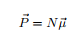Where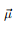is average dipole moment per molecule and N is number of molecules per unit volume.
Also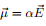where α is a constant of proportionality called polarizability

In Polar dielectric materials, when the external electric field is applied all dipoles tend to align in the field direction and hence net dipole moment develops across the dielectric material.
In non polar dielectric materials dipoles are induced due to the applied electric field which results in the net dipole moment in the dielectric material in the direction of the applied field.
As the polarization measures the additional flux density arising from the presence of the material as compared to free space it has the same unit as D and is related to it as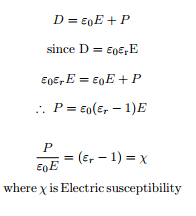Types of Polarization
Polarization takes place through four different mechanisms. They are
1. Electronic polarization.
2. Ionic polarization.
3. Orientation polarization.
4. Space charge polarization.

The net polarization of the material is due to the contribution of all four polarization mechanisms.
P = Pe + Pi + Po + Ps

(1) Electronic polarization:
This occurs through out the dielectric material and is due to the separation of effective centers of positive charges from the effective centers of negative charges in atoms or molecules of dielectric material due to applied electric field. Hence dipoles are induced within the material. This leads to the development of net dipole moment in the material and is the vector sum of dipole moments of individual dipoles.
The electric dipole moment is given by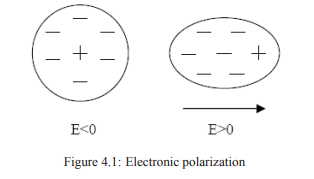µe ∝ E

µe = αeE

Where αe = 4πε0R3 electronic polarizability. Therefore
µe = 4πε0R3E

Electronic Polarization Pe is given by

Pe = Nµe = NαeE where N is number of atoms per m3

(2) Ionic polarization:
This occurs in ionic solids such as sodium chloride etc. Ionic solids possess net dipole moment even in the absence of external electric field. But when the external electric field is applied the separation between the ions further increases. Hence, the net dipole moment of the material also increases.
It is found that the ionic dipole moment is also proportional to the applied field strength.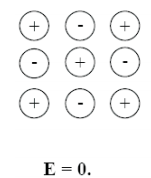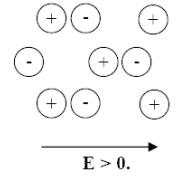Figure 4.2: Ionic polarization

hence µi = αiE
where αi is ionic polarisability
Ionic Polarization is given by ionic dipole moment per unit volume.Hence
P= Nµi

where N is the number of atoms per unit volume.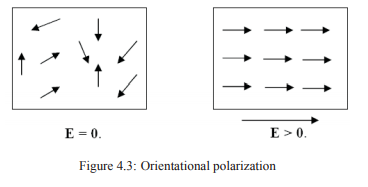(3) Orientation Polarization:
This occurs in polar dielectric material, which possess permanent electric dipoles. In polar dielectrics the dipoles are randomly oriented due thermal agitation. Therefore net dipole moment of the material is zero. But when the external electric field is applied all dipoles tend to align in the field direction. Therefore dipole moment develops across the material. This is referred to as orientation polarization (P0). Orientation polarization depends on temperature. Higher the temperature more will be the randomness in dipole orientation thereby reducing the dipole moment.

The orientation polarizability is given by α0 = µ2/3kT , where ‘k’ is Boltzman constant, T is absolute temperature and µ is permanent dipole moment.

(4) Space charge polarization:
This occurs in materials in which only a few charge carriers are capable of moving through small distances. When the external electric field is applied these charge carriers move. During their motion they get trapped or pile up against lattice defects. These are called localized charges. These localized charges induce their image charge on the surface of the dielectric material. This leads to the development of net dipole moment across the material. Since this is very small it can be neglected. It is denoted by Ps.

Offer running on EduRev: Apply code STAYHOME200 to get INR 200 off on our premium plan EduRev Infinity!

## Engineering Physics - Notes, Videos, MCQs & PPTs

41 videos|48 docs|9 tests

,

,

,

,

,

,

,

,

,

,

,

,

,

,

,

,

,

,

,

,

,

;